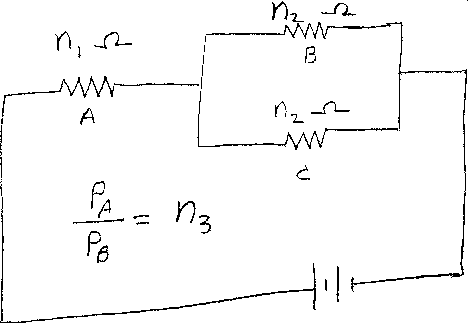Problem B9: Consider the circuit shown above that has three resistors, which we lable as A, B, and C. Resistor A has a resistance of n1 Ohms. Resistors B and C have the same resistance, which is n2 Ohms. What is the ratio of the power dissipated in resistor A, PA, to the power dissipated in resistor B, PB? PA / PB = n3. What is n3?n1 = n2 = Input n3:
If you are currently in my class, you can record your grade by entering your name and student ID number (without the leading zeros) below and clicking on "record grade".
 First Name = Last Name = ID = Problem: